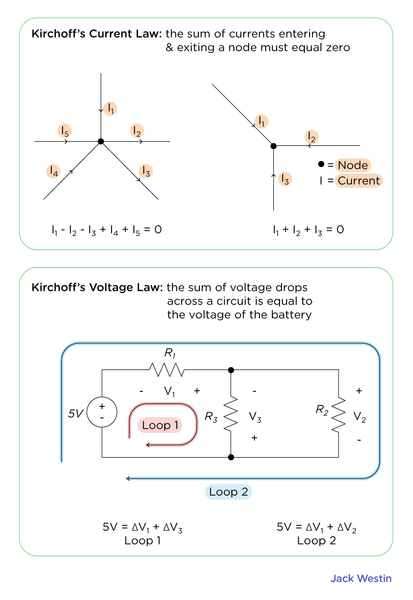Complimentary 1-hour tutoring consultation

MCAT Content / Circuit Elements / Current I Dq Dt Sign Conventions Units

### Current I = ΔQ/Δt, sign conventions, units

Topic: Circuit Elements

Current (I) is the measurement of the amount of moving charge passing through a cross-section over a period of time. I = ΔQ/Δt.

While in reality, it’s normally the electrons that are moving, we assume current is the flow of positive charges by convention.

The SI unit of current is Ampere (A)

To measure current we can measure the change in charge (ΔQ) divided by change in time (Δt):

I (A) = ΔQ (C) / Δt (s)

To increase the current, we can increase the amount of charge, the charge value, the cross-section for more charge to pass through, and the velocity of the charge.Practice Questions

MCAT Official Prep (AAMC)

Physics Question Pack Passage 4 Question 28

Sample Test C/P Section Passage 2 Question 9

Sample Test C/P Section Passage 7 Question 37

Practice Exam 1 C/P Section Passage 10 Question 56

Key Points

• I = ΔQ/Δt, the current is the amount of charge moving through a cross-section, over a period of time.

• Current is the flow of positive charge.

• Unit of current I is Ampere (A)

Key Terms

cross-section: a surface or shape exposed by making a straight cut through something, especially at right angles to an axis.

current: is the amount of charge moving through a cross-section, over a period of time.

Billing Information